# Improve Searching in Linked List

## Improve Searching in Linked List with Examples

In our previous article, we discussed linear search in the linked list. In this article, we will see how we can improve the linear search method in the linked list with examples.

##### Improve Searching in the linked list

For improving Linear Search, there are two methods:

• Transposition

We have seen these two methods in array topics. So these procedure is for improving. So that next time, when you search for the same key it can be found in less time.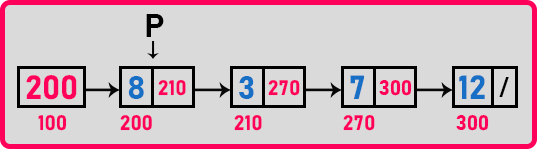Transposition is a method where we interchange the value with the previous value. So, if suppose we are searching for ‘12’ then ‘12’ will be interchanged with the previous value that is with ‘7’. And the second method moved to head means the key value should be brought in the beginning so that next time the search for the same key it is found just in one comparison.

In the linked list which is suitable? Transposition we don’t use because we avoid the movement of data in the linked list. We prefer the movement of nodes. So, this better we take out the node ‘12’ and added it to the front of the linked list. So next time when we search it can be found just in one comparison. We will explain to you how it can be done.

##### The procedure of Move to Head method:

Let us look at the code for linear search along with the move to head. Suppose ‘12’ is the key we want to bring at the beginning of the linked list.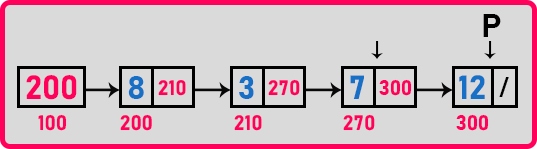So, for this, what are the steps we have to perform? First, we will move the pointer ‘p’ to the key node. In this case, ‘12’ is the key. So which node do we have to modify? We have to modify the key node. Then we have to modify the previous node also which is pointing to the key node. In this case, ‘7’ is the previous node. So, for that, we should have a pointer on the previous node.

How to get a pointer on the previous node? In the linked list, we can get a pointer on the next node but how to point the previous node? So let us get a pointer on the previous node. Let us see the procedure.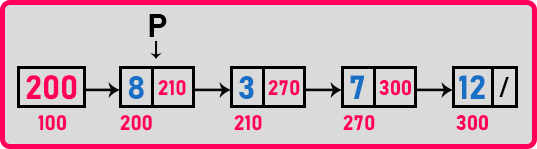Initially, ‘p’ is pointing on the first node. Now here we should have one more pointer ‘q’. It will follow pointer ‘p’. We will call ‘q’ as a tail pointer. ‘q’ is null when ‘p’ is pointing on the first node. We have to check every node whether its data is equal to key, if not move ‘p’ to next and if we found then stop there. Now let us see the steps.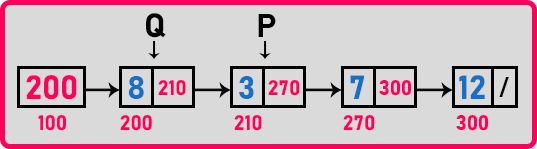Now, ‘p’ is pointing to the next node i.e. ‘3’. So ‘q’ has to follow ‘p’. ‘q’ will point on the previous node where ‘p’ is pointing i.e. ‘8’. We will check for ‘if (p->data == key)’. ‘p’ is not pointing to the key element. So move ‘p’ as well as ‘q’.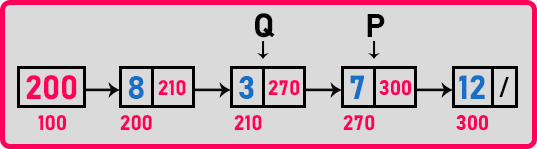Here also ‘p’ is not pointing to the key element. So move ‘q’ and ‘p’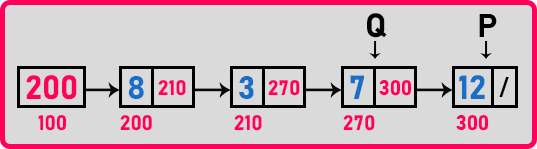Here ‘p’ is pointing to the key element which is ‘12’. Now we have to bring this node to the beginning of the linked list.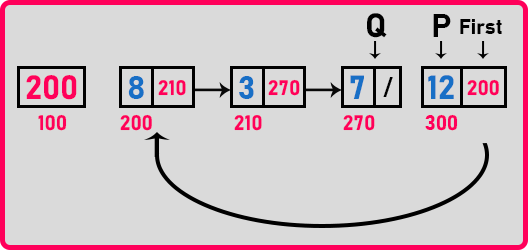So as seen in the diagram, we have modified 2 nodes. We modify the ‘12’ node to point on the first node and also modify the previous node that is ‘7’ to point on null as there is no more node, it is the last node in the linked list. Now let us write a function for this.

##### Function for Improve Linear Search:
```struct Node* LSearch(struct Node *p, int key)
{
struct Node *q;
while (p != NULL)
{
if (key == p->data)
{
q->next = p->next;
p->next = first;
first = p;
return p;
}
q = p;
p = p->next;
}
return NULL;
}
```

Now let us write the full program.

##### Program of Improve Linear Search in a Linked List using C Language:
```#include <stdio.h>
#include <stdlib.h>

struct Node
{
int data;
struct Node *next;
}   *first = NULL;

void Create(int A[], int n)
{
int i;
struct Node *t, *last;
first = (struct Node *) malloc(sizeof (struct Node));
first->data = A;
first->next = NULL;
last = first;

for (i = 1; i < n; i++)
{
t = (struct Node *) malloc(sizeof (struct Node));
t->data = A[i];
t->next = NULL;
last->next = t;
last = t;
}
}

struct Node* LSearch(struct Node *p, int key)
{
struct Node *q;
while (p != NULL)
{
if (key == p->data)
{
q->next = p->next;
p->next = first;
first = p;
return p;
}
q = p;
p = p->next;
}
return NULL;
}

int main()
{
struct Node *temp;
int A[] = { 8, 3, 7, 12 };
Create(A, 4);
temp = LSearch(first, 12);
printf(" %d", temp->data);
return 0;
}
```
###### Output:In the next article, I am going to discuss Inserting a new node in a linked list using C Language with Examples. Here, in this article, I try to explain Improve Searching in a linked list using C Language with Examples and I hope you enjoy this Improve Searching in a linked list with Examples article.